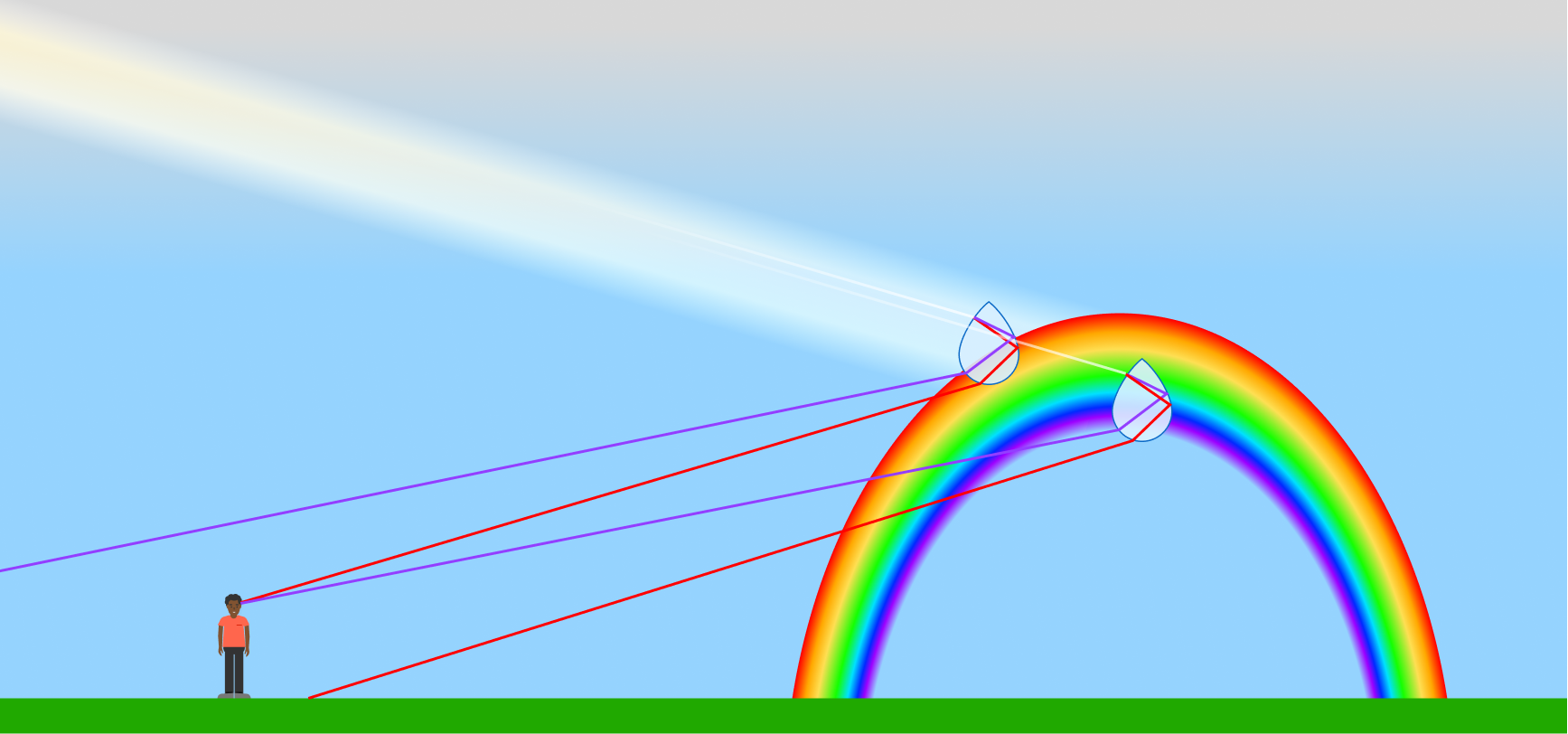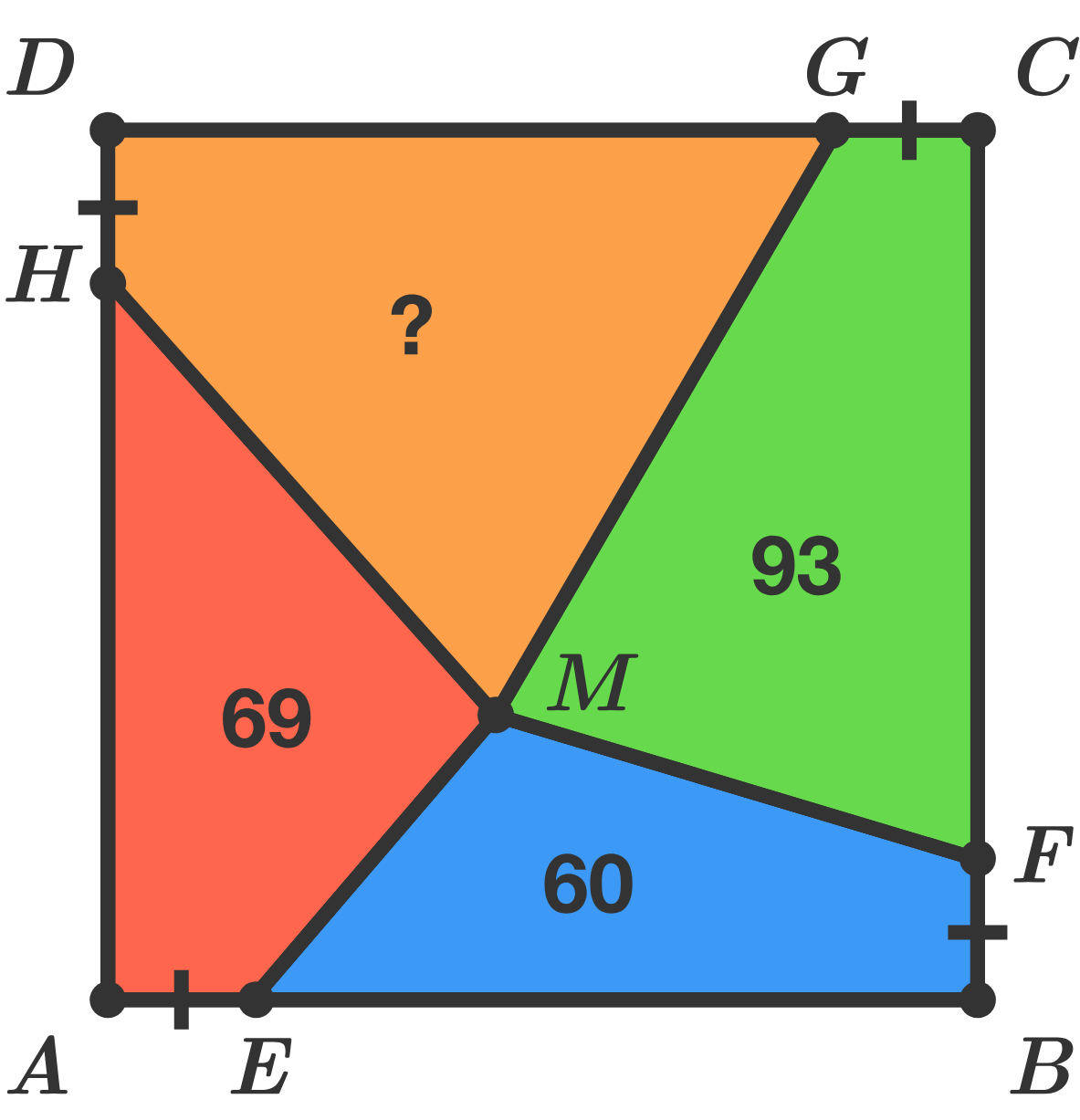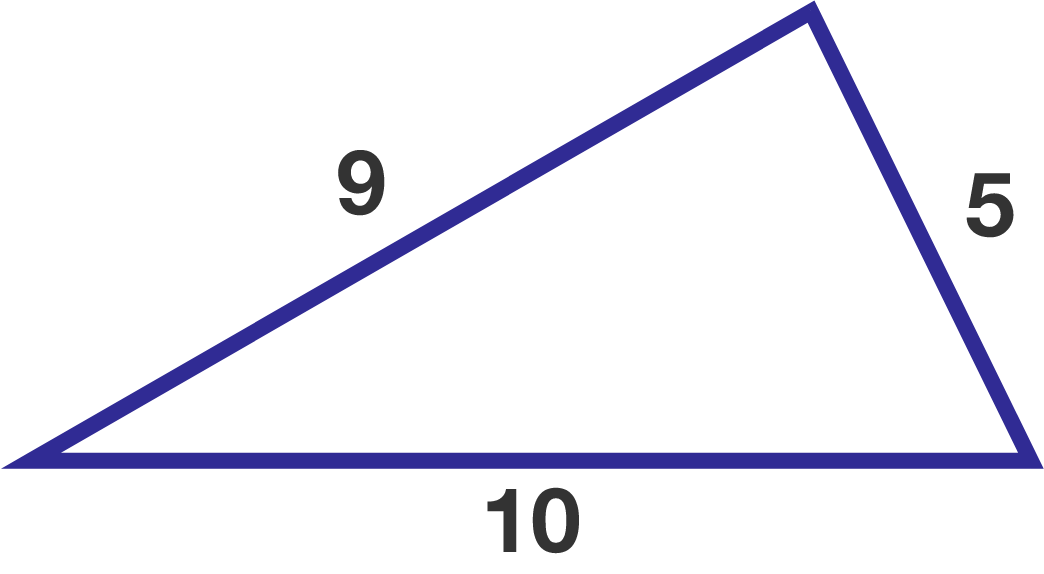# Problems of the Week

Contribute a problem

# 2018-08-27 Intermediate

When sunlight passes through water droplets in the atmosphere, some of the refracted light is reflected. Because different colors bend by different angles, we see a circular arc of different colors—a rainbow.

At what time of day does a rainbow appear the tallest when viewed from the ground?$ABCD$ is a square and $AE=BF=CG=DH.$

What is the area of the orange quadrilateral $DHMG?$A teacher wrote a number on the board and asked the students to tell about the divisors of the number one by one.

• The $1^\text{st}$ student said, "The number is divisible by 2."
• The $2^\text{nd}$ student said, "The number is divisible by 3."
• The $3^\text{rd}$ student said, "The number is divisible by 4."
• $\quad \quad \vdots$
• The $30^\text{th}$ student said, "The number is divisible by 31."

The teacher then said that exactly two consecutive students were incorrect.

Which two students were incorrect?

If the $m^\text{th}$ and $(m+1)^\text{th}$ students spoke wrongly, then enter $m.$

$a !+ b !+c != d !,$ where $a, b, c, d$ are all positive integers.

What is the number of possible solutions $(a, b, c, d)?$

Suppose you inscribe a smaller triangle in the triangle below such that its three vertices are on different sides of the given triangle.

What is the smallest possible perimeter of the inscribed triangle?×